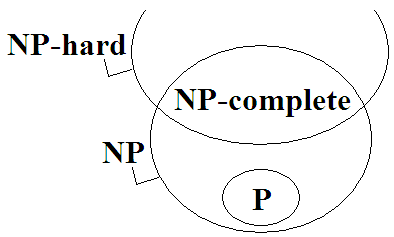Algorithm Analysis

Algorithm Analysis

Time Complexity

```BUBBLESORT(A, N)                  | steps
1 for i ← 0 to N-1 do             | N
2     for j ← i to N-i-1 do       | N(N-1)/2
3         if A[j] < A[j+1] then   | N(N-1)/2
4             temp ← A[j]         | at most N(N-1)/2
5             A[j] ← A[j+1]       | at most N(N-1)/2
6             A[j+1] ← temp       | at most N(N-1)/2
```
```total = N + 5N(N-1)/2
= N + 2.5N² - 2.5N
= 2.5N² - 1.5N
= O(N²)
```

```               | time*    | space
---------------+----------+--------
bubble sort    | O(N²)    | O(N)
insertion sort | O(N²)    | O(N)
merge sort     | O(NlogN) | O(N)
quicksort      | O(N²)    | O(N)
heapsort       | O(NlogN) | O(N)
counting sort  | O(N+R)   | O(N+R)

*worst-case
```

```best-case complexity：找到最佳的輸入順序。時間複雜度的最小值。
worst-case complexity：找到最壞的輸入順序。時間複雜度的最大值。
average-case complexity：統計每一種輸入順序。時間複雜度的平均值。
```

Time Complexity of Recurrence Relation

Master Theorem

Akra–Bazzi Method

Amortized Analysis

multipop stack

```1. push(x)      存入一筆資料。如果stack是滿的就馬上停止。
worst time O(1)
2. pop()        取出一筆資料。如果stack是空的就馬上停止。
worst time O(1)
3. multipop(k)  連續取出k筆資料。如果stack是空的就馬上停止。
worst time O(min(n,k)) 當stack有n個元素
```

multipop(k)的時間複雜度是O(min(n,k))，n是當下的資料數量，k是欲取出的資料數量。因為n和k最大可到N，所以時間複雜度是O(N)。

multipop(k)最多實施N次，總時間複雜度是O(N²)。

N次操作，最多存入N筆資料。即是N次push()。

N次操作，最多取出N筆資料。無論pop()或multipop(k)。

N次操作，最多存取2N筆資料。無論哪種操作。

N次操作，總時間複雜度是O(2N) = O(N)。

Amortized Analysis：步驟數量不固定，採取累計的方式。

Smoothed Analysis：分析輸入順序有多少機率是整齊的、多少機率是雜亂的。

Competitive Analysis：步驟數量、輸入順序不固定，運用機率來分析時間複雜度。

Complexity Notation

O

Ω下限。O上限。Θ同時滿足下限與上限（剛剛好）。

```f(n) = Ω(g(n))  ⟺  f(n) ≥ c ⋅ g(n) for any constant c
f(n) = O(g(n))  ⟺  f(n) ≤ c ⋅ g(n) for any constant c
f(n) = Θ(g(n))  ⟺  f(n) = c ⋅ g(n) for any constant c
```

o

ω下級。o上級。

```f(n) = ω(g(n))  ⟺  f(n) > c ⋅ g(n) for all constant c
f(n) = o(g(n))  ⟺  f(n) < c ⋅ g(n) for all constant c
```

Õ

```Õ(g(x)) = O( g(x) logᵏg(x) ) = O( g(x) polylog g(x) )
polylog(x) = logᵏx
```

log*

α(n)

Ackermann function f(n,n)的反函數。實務上與常數無異。

O(𝓜(n))

O(nω)

Type of Computation

Model of Computation

Turing Machine

brainfuck程式語言有點類似此模型。

Word RAM

C程式語言有點類似此模型。

NP-completeP問題

```「找出一群數字當中最大的數字」是P問題。
```

P的全名是Polynomial time，定義源自於「自動機理論」，頗複雜，此處省略之。通常以「P」表示所有P問題構成的集合。

NP問題

```「找出一張圖的一條Hamilton Path」是NP問題。

```
```「找出一張圖成本最小的那條Hamilton Path」不是NP問題。

```

NP的全名是Non-deterministic Polynomial time，定義源自於「自動機理論」，頗複雜，此處省略之。通常以「NP」表示所有NP問題構成的集合。

NP-complete問題

NP-complete問題的各種特例，涵蓋了所有NP問題。只要有辦法解決NP-complete問題，就有辦法解決NP問題。

Complete的意義為：能夠代表整個集合的子集合。舉例來說，它就像是一個線性空間（linear space）的基底（basis）。

```「判斷一張圖是否存在Hamilton Path」已被證明是NP-complete問題。
```

NP-hard問題

NP-hard問題可能是：甲、NP-complete問題（是NP問題），乙、超出NP問題的複雜度，是更難的問題。

```「找出一張圖成本最小的Hamilton Path」是NP-hard問題。

```

Weakly NP-complete

Pseudo-polynomial Time

```「一堆整數，從中挑出一些，總和為0」是NP-complete問題。
```

R其實是2ᴷ，O(RNNK)其實是O(2ᴷNNK)，只不過K很小。外觀是多項式時間，實際執行也是多項式時間，本質卻是指數時間，因此稱作「偽多項式時間」。

Weakly NP-complete問題、Strongly NP-complete問題

NP-complete問題，可以達到偽多項式時間者，稱作Weakly NP-complete問題；反之稱作Strongly NP-complete問題。

```「一堆整數，從中挑出一些，總和為0」是Weakly NP-complete問題。
「判斷一張圖是否存在Hamilton Path」是Strongly NP-complete問題。
```

NP-complete、NP-hard並不是好的分類方式，導致事情很複雜。我真的不想介紹NP-complete。但是教科書都這樣教，我也只好配合一下。

P versus NP

P = NP ?

Dijkstra演算法是確定性演算法，每個步驟都是確定的、固定的，所以時間複雜度上限和下限是一樣的，沒有特地討論的必要。

「挑選一個」與「窮舉全部」的差別，這就是困難所在之處。

P = NP ?

P = NP問題的困難之處，在於證明時間複雜度下限。必須證明NP問題的時間複雜度下限，到底是和P一樣是多項式時間、或者是和NP-complete一樣是指數時間。超級難的！

Complexity Class

Complexity Class

```PTIME / PSPACE / EXPTIME / EXPSPACE  時間用量、空間用量
NC⁰ / AC⁰ / ACC⁰                     邏輯閘用量
```
```P     判定問題
#P    計數問題
PP    機率判定問題
```

Approximation Algorithm

```APX   有多項式時間演算法
近似到 MINOPT⋅n 或者 MAXOPT⋅n 對於一個n

PTAS  有偽多項式時間演算法 (n的多項式，ε視作定值)
近似到 MINOPT⋅(1+ε) 或者 MAXOPT⋅(1-ε) 對於所有ε

FPTAS 有多項式時間演算法 (1/ε和n兩種變數構成的多項式)
近似到 MINOPT⋅(1+ε) 或者 MAXOPT⋅(1-ε) 對於所有ε
```

Randomized Algorithm

```BPP   有多項式時間演算法
出錯機率不超過1/2，正確機率至少1/2。
執行 k 次，投票表決，讓出錯機率不超過 (1/2)ᵏ

RP    有多項式時間演算法
當答案為Yes，有1/2機率出錯。
當答案為No，絕對不會出錯。
執行 k 次，投票表決，讓出錯機率降為 (1/2)ᵏ

ZPP   有多項式時間演算法
要嘛回答正確答案，要嘛不知道答案，機率各1/2。
```

Algorithm Class

Offline Algorithm / Online Algorithm

「離線演算法」是一口氣輸入所有資料之後，才能開始運行的演算法。例如Bubble Sort。

「在線演算法」是不需等待所有資料到達，就可以分時分段處理輸入的演算法。例如Insertion Sort。

Static Algorithm / Dynamic Algorithm

「靜態演算法」是無法隨時修改、增加、減少原本的輸入資料，無法隨時查詢輸出的演算法。例如Dijkstra's Algorithm。

「動態演算法」是可以隨時修改、增加、減少原本的輸入資料，可以隨時查詢輸出的演算法。例如Binary Search Tree。

Exact Algorithm / Approximation Algorithm

「精確演算法」是計算結果絕對正確的演算法。

「近似演算法」是計算結果擁有誤差的演算法。

Deterministic Algorithm / Randomized Algorithm

「確定性演算法」是計算步驟順序固定的演算法。

「隨機化演算法」是計算步驟順序隨意的演算法。

Open Problem

「懸案」。目前還沒有人知道正確答案的問題。

```Open Problem Garden
The Open Problems Project
Egres Open
10000个科学难题·数学卷
The Princeton Companion to Mathematics、普林斯頓數學指南
```

Intractable Problem

「難題」。難以設計快速的演算法。

```Tractability: Practical Approaches to Hard Problems
```

```一、數學規劃。請參考「Integer Programming」。

```

```[Picking]
Boolean Satisfiability Problem：設定真假值，使邏輯計算式結果為真。

[Partitioning]
Partition Problem：一群數字，分成兩群，讓兩群總和一樣多。
Integer Factorization：一個數字乘積，拆散成數字。

[Packing]
Packing Problem：使用特定幾何圖形，互不重疊，盡量填滿空間。
Knapsack Problem：一個容器，一堆物品，盡量放進所有物品。
Bin Packing Problem：一堆容器，一堆物品，使用最少容器，放進所有物品。
Box Stacking Problem：3D無蓋箱子堆疊。

[Covering]
Covering Problem：使用特定幾何圖形，覆蓋空間，數量盡量少。
Set Cover Problem：一堆集合，各自包含某些元素。用最少個集合，涵蓋所有元素。
Steiner Tree Problem：使用線條，連接所有地點，長度盡量短。
Facility Location Problem：選定P點，連接所有地點，成本盡量小。

[Scheduling]
Hamilton Path：拜訪所有景點的最短路線。
Vehicle Routing Problem：從起點出發多次，拜訪所有景點的最短路線。
Scheduling Problem：工廠進行生產製造、規劃最佳流程。
Graph Coloring：地圖相鄰區塊填入不同顏色，顏色種類盡量少。
Chess Problem：給定棋盤盤面，找到贏棋下法。
```